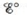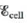# A chemist wishes to determine the concentration of CrO 4 2− electrochemically. A cell is constructed consisting of a saturated calomel electrode (SCE; see Exercise 115) and a silver wire coated with Ag 2 CrO 4 . The value for the following half-reaction is 0.446 V relative to the standard hydrogen electrode: Ag 2 CrO 4 + 2 e − → 2 Ag + CrO 4 2 − a. Calculate and ∆ G at 25°C for the cell reaction when [CrO 4 2− ] = 1.00 mol/L. b. Write the Nernst equation for the cell. Assume that the SCE concentrations are constant. c. If the coated silver wire is placed in a solution (at 25°C) in which [CrO 4 2− 1] = 1.00 × 10 −5 M , what is the expected cell potential? d. The measured cell potential at 25°C is 0.504 V when the coated wire is dipped into a solution of unknown[CrO 4 2− ]. What is [CrO 4 2− ] for this solution? e. Using data from his problem and from Table 17-1, calculate the solubility product ( K sp ) for Ag 2 CrO 4 .### Chemistry: An Atoms First Approach

2nd Edition
Steven S. Zumdahl + 1 other
Publisher: Cengage Learning
ISBN: 9781305079243

#### Solutions

Chapter
Section### Chemistry: An Atoms First Approach

2nd Edition
Steven S. Zumdahl + 1 other
Publisher: Cengage Learning
ISBN: 9781305079243
Chapter 17, Problem 152CP
Textbook Problem
10 views

## A chemist wishes to determine the concentration of CrO42− electrochemically. A cell is constructed consisting of a saturated calomel electrode (SCE; see Exercise 115) and a silver wire coated with Ag2CrO4. Thevalue for the following half-reaction is 0.446 V relative to the standard hydrogen electrode: Ag 2 CrO 4   +   2 e −   →   2 Ag   +   CrO 4 2 − a. Calculateand ∆G at 25°C for the cell reaction when [CrO42−] = 1.00 mol/L.b. Write the Nernst equation for the cell. Assume that the SCE concentrations are constant.c. If the coated silver wire is placed in a solution (at 25°C) in which [CrO42−1] = 1.00 × 10−5 M, what is the expected cell potential?d. The measured cell potential at 25°C is 0.504 V when the coated wire is dipped into a solution of unknown[CrO42−]. What is [CrO42−] for this solution?e. Using data from his problem and from Table 17-1, calculate the solubility product (Ksp) for Ag2CrO4.

(a)

Interpretation Introduction

Interpretation: The standard cell potential Eο and standard Gibbs free energy ΔGο of the given cell are to be calculated. The Nernst equation for the cell is to be stated. The molar concentration of [CrO42] for this solution and solubility product (Ksp) for Ag2CrO4 is to be calculated.

Concept introduction: Cell potential is defined as the measure of energy per unit charge available from the redox reaction to carry out the reaction. Gibbs free energy is a thermodynamic quantity that is used to calculate the maximum work of reversible reaction performed by a system. It is equal to the difference between the enthalpy and the product of entropy at absolute temperature.

To determine: The standard cell potential Eο and standard Gibbs free energy ΔGο at 2οC .

### Explanation of Solution

Explanation

The half cell reactions are,

Anode:   HgHg2++2e        Eanodeο=0.242 V

Cathode: 2Ag++2e2Ag   Ecathodeο=0.446 V

Where,

• Eanodeο is the standard reduction potential of anode.
• Ecathodeο is the standard reduction potential of cathode.

Cell potential of the reaction is the difference in voltage between the cathode and anode. The cell potential is calculated by using the expression,

Eο=Eο(cathode)Eο(anode) .

Substitute the value of standard reduction potential of anode and cathode in the above expression to calculate the value of cell potential of the reaction.

Eο=Eο(cathode)Eο(anode)=0.446 V0.242 V=0.204 V_

Hence, the standard cell potential of reaction is 0

(b)

Interpretation Introduction

Interpretation: The standard cell potential Eο and standard Gibbs free energy ΔGο of the given cell are to be calculated. The Nernst equation for the cell is to be stated. The molar concentration of [CrO42] for this solution and solubility product (Ksp) for Ag2CrO4 is to be calculated.

Concept introduction: Cell potential is defined as the measure of energy per unit charge available from the redox reaction to carry out the reaction. Gibbs free energy is a thermodynamic quantity that is used to calculate the maximum work of reversible reaction performed by a system. It is equal to the difference between the enthalpy and the product of entropy at absolute temperature.

To determine: The Nernst equation for the cell.

(c)

Interpretation Introduction

Interpretation: The standard cell potential Eο and standard Gibbs free energy ΔGο of the given cell are to be calculated. The Nernst equation for the cell is to be stated. The molar concentration of [CrO42] for this solution and solubility product (Ksp) for Ag2CrO4 is to be calculated.

Concept introduction: Cell potential is defined as the measure of energy per unit charge available from the redox reaction to carry out the reaction. Gibbs free energy is a thermodynamic quantity that is used to calculate the maximum work of reversible reaction performed by a system. It is equal to the difference between the enthalpy and the product of entropy at absolute temperature.

To determine: The expected cell potential.

(d)

Interpretation Introduction

Interpretation: The standard cell potential Eο and standard Gibbs free energy ΔGο of the given cell are to be calculated. The Nernst equation for the cell is to be stated. The molar concentration of [CrO42] for this solution and solubility product (Ksp) for Ag2CrO4 is to be calculated.

Concept introduction: Cell potential is defined as the measure of energy per unit charge available from the redox reaction to carry out the reaction. Gibbs free energy is a thermodynamic quantity that is used to calculate the maximum work of reversible reaction performed by a system. It is equal to the difference between the enthalpy and the product of entropy at absolute temperature.

To determine: The molar concentration of [CrO42] for the given Answer.

(e)

Interpretation Introduction

Interpretation: The standard cell potential Eο and standard Gibbs free energy ΔGο of the given cell are to be calculated. The Nernst equation for the cell is to be stated. The molar concentration of [CrO42] for this solution and solubility product (Ksp) for Ag2CrO4 is to be calculated.

Concept introduction: Cell potential is defined as the measure of energy per unit charge available from the redox reaction to carry out the reaction. Gibbs free energy is a thermodynamic quantity that is used to calculate the maximum work of reversible reaction performed by a system. It is equal to the difference between the enthalpy and the product of entropy at absolute temperature.

To determine: The solubility product (Ksp) for Ag2CrO4 .

### Still sussing out bartleby?

Check out a sample textbook solution.

See a sample solution

#### The Solution to Your Study Problems

Bartleby provides explanations to thousands of textbook problems written by our experts, many with advanced degrees!

Get Started

Find more solutions based on key concepts
Both carbohydrates and protein have 4 calories per gram. T F

Nutrition: Concepts and Controversies - Standalone book (MindTap Course List)

Why does the expansion of a stars envelope make it cooler and more luminous?

Horizons: Exploring the Universe (MindTap Course List)

The head of a grass string trimmer has 100 g of cord wound in a light, cylindrical spool with inside diameter 3...

Physics for Scientists and Engineers, Technology Update (No access codes included)

How many accelerators does a car have? What are they?

Foundations of Astronomy (MindTap Course List)

How was bathymetry accomplished in years past? How do scientists do it now?

Oceanography: An Invitation To Marine Science, Loose-leaf Versin